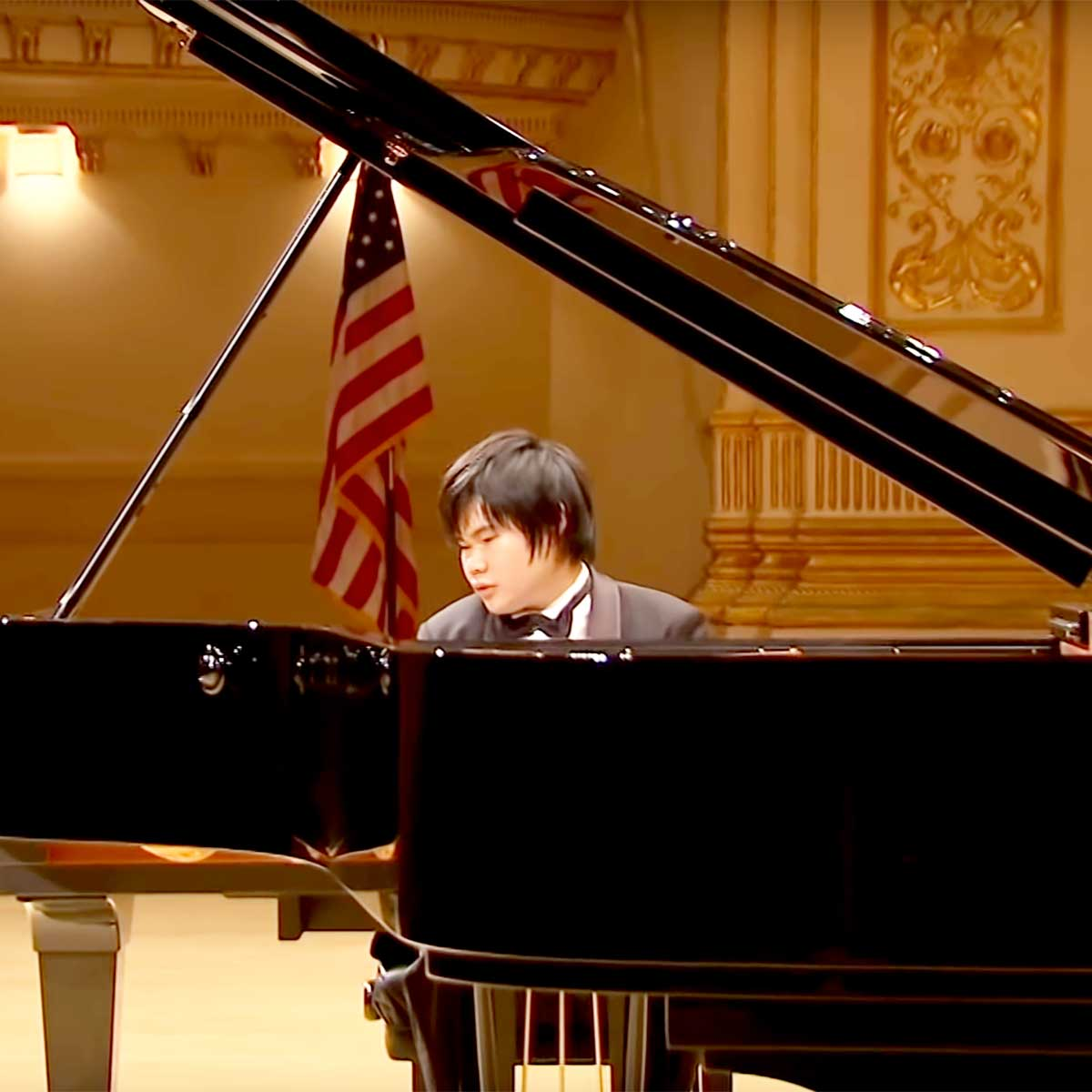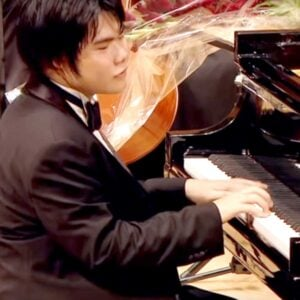1 Music Sheets

• ###### Born:

13th September 1988

• ###### Genres:

Nobuyuki Tsujii (辻井 伸行, Tsujii Nobuyuki) (also known as Nobu Tsujii) is a Japanese pianist and composer. He was born blind due to microphthalmia, and his exceptional musical talent has propelled him to become a world renowned artist. Tsujii performs extensively, with a large number of conductors and orchestras, and has received critical acclaims as well as notices for his unique techniques for learning music and performing with an orchestra while being unable to see.

Credit: Wikipedia

## Artist's Music Sheets

•8 w y Y o 8 w y Y o s d [D8o] w y Y [os] D d s [O8d] W y Y i d D [7og] w y Y i g h H [D8h] w t Y [Dos] s s [tfhl] u o [Pl] [Lf] l h [Jqg] W [Ht] i O [Yh] [Gy] I [pz] s [Gj] l [wl] y i o [ak] s d [D8o] w y Y [os] D d s [O8d] W y Y i d D [7og] w y Y i g h H [D8h] w t Y [Dos] s s [tfhl] u o [Pl] [Lf] l h [Jqg] W [Ht] i O H h g [wh] t Y o Z [wz] r y i k [D8hl] w t Y o s s [Hql] t i [OJh] [Hsg] J l [(Dhl] t [Yh] o s h H h [yh] i O g w r y i g h g [D8s] w t [Ydg] [Doh] s s [Hql] t i [OJh] [Hsg] J l [(Dhl] t [Yh] o s h H h [Gy] I [pz] s [Gj] l [wl] y i o s k l z l [wk] y i o a d g h k z v o o [8fh] w t [udg] [osf] g h [7oah] w [rd] y [oa] u [6sf] 0 r [td] [us] d f [0of] r [ua] I o o o [qp] e t [ij] [qah] w [rg] y [0ah] w [rf] [yd] [es] t [us] [oa] [9ip] e t y [I9p] e a t s y [wipsd] t y i [wyioad] o o [8fh] w t [udg] [osf] g h [7oah] w [rd] y [oa] o o [^Pd] w E [yf] [og] f d [eg] t [is] p i s [WHl] t i [OJh] [Hsg] J l [wsfhl] t [uh] o [sf] s s [wpsg] y i g [woaf] y i d [8s] w t u [osfhl] s s [qjl] t i [phk] [sgj] k l [0sfhl] t [uh] o [sf] s s [9psg] e t [if] [wad] [rf] [yg] [ij] [P8dgh] w E i [wsfh] E t u s s [qjl] t i [phk] [sgj] k l [0sfhl] t [uh] o s s [9d] e t [yf] [IG] f d [wpsh] y i [ps] [wyioah] o o [8fh] w t [udg] [osf] g h [7oah] w [rd] y [oa] u u [6sf] 0 r [td] [us] d f [0of] r [ua] I o o o [qp] e t [ij] [qah] w [rg] y [0ah] w [rf] [yd] [es] t [us] [oa] [9ip] e t y [I9p] e a t s y [wipsd] t y i [wyioad] o [8fh] w t [udg] [osf] g h [7oah] w [rd] y [oa] o o [^Pd] w E [yf] [og] f d [eg] t [is] p i s [WHl] t i [OJh] [Hsg] J l [wsfhl] t [uh] o [sf] s s [wyipsg] g [wyioaf] d [8s] w t u [of] o [qp] e t [ij] [qah] w [rg] y [0ah] w [rf] [yd] [es] t [us] [os] [9ipsg] e t y [ips] i i [WOisg] t y i [Os] g [wpsg] y i p s p [ig] y [wiaf] y i o [ad] o i y [8uos] w y u o y u o [8ups] e u i p u i p [8wyuos]

Level: 7
Length: 03:35
Intermediate